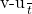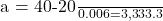## A 0.2 kg baseball moving with a velocity of 20 m/s is struck by a bat. The baseball reverses its direction and moves with a velocity of 40 m

Question

A 0.2 kg baseball moving with a velocity of 20 m/s is struck by a bat. The baseball reverses its direction and moves with a velocity of 40 m/s . What is the average force exerted on the ball if the bat is in contact with the ball for 0.006 ?

in progress 0
1 month 2021-08-12T13:41:22+00:00 1 Answers 0 views 0

1. Average force is 666.67 N

Explanation:

Given that :

m = Mass of the baseball = 0.2 kg

u = Initial velocity = 20 m/s

v = Final velocity = 40 m/s

t = Time taken for change in velocity = 0.006 s

We know:

Force exerted = mass × acceleration = m ×a

Acceleration can be found by means of dividing the change in velocity measured in m/s by the time taken in seconds.

a ==m/s²

Now we have to find the force using the formula, F = mass × acceleration as,

F = 0.2 kg ×3333.3 ms⁻² = 666.67 N